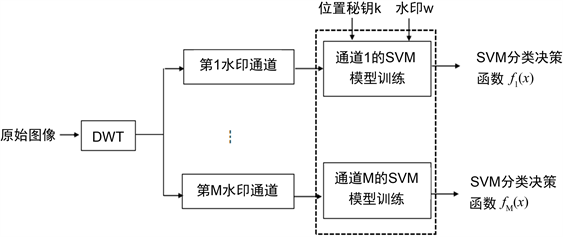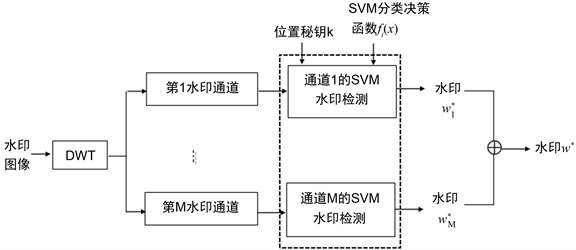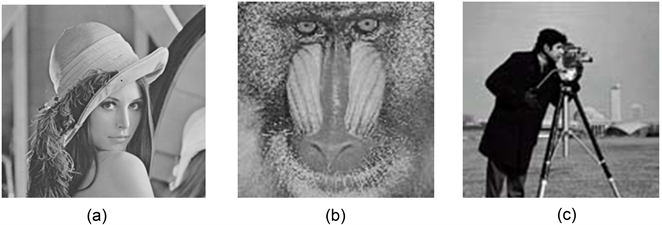# 基于SVM的多通道鲁棒图像零水印算法A Robust Image Zero-Watermarking Algorithm Based on Multi-Channel and SVM

DOI: 10.12677/JISP.2020.91008, PDF, HTML, XML, 下载: 210  浏览: 326  科研立项经费支持

Abstract: This paper proposes a robust image zero-watermarking scheme based on a multi-channel watermarking model and Support Vector Machine (SVM). In the scheme, the DWT domain of the original image is divided into many non-overlapping watermarking channels, and SVM training sets of all watermarking channels are built based on robust watermark and DWT coefficients decided by a logistic chaos key. By SVM training, we obtain decision functions of all watermarking channels. During the watermark detecting stage, watermarks of all watermarking channels are extracted by the decision functions, and the final robust watermark is worked out by an estimation function. With the proposed algorithm, the watermarked images are perfect without any distortion since we don’t embed any information into the original image, and they can resist various intensive watermarking attacks.

1. 引言

2. 支持向量机

$w•x+b=0$ (1)

$\mathrm{max}\mathrm{min}L\left(w,b,a\right)=\frac{1}{2}{‖w‖}^{2}-\underset{i=1}{\overset{l}{\sum }}{a}_{i}{y}_{i}\left[w•{x}_{i}-b\right]+\underset{i=1}{\overset{l}{\sum }}{a}_{i}$ (2)

$f\left(x\right)=\mathrm{sgn}\left({w}^{*}•x+{b}^{*}\right)$ (3)

3. 基于SVM的多通道零水印方案

3.1. 多通道分类决策函数生成Figure 1. Building classification decision function

3.2. 图像水印检测

${w}_{i}^{*}\left(j\right)={f}_{i}\left({C}_{i}^{*}\left(j\right)\right),\text{\hspace{0.17em}}\text{\hspace{0.17em}}i=1,2,\cdots ,M;j=1,2,\cdots ,N$ (4)Figure 2. Watermark detection

${w}^{*}\left(i\right)=\mathrm{sgn}\left(\underset{k=1}{\overset{M}{\sum }}{w}_{k}^{*}\left(i\right)\right),\text{\hspace{0.17em}}\text{\hspace{0.17em}}\text{\hspace{0.17em}}i=1,2,\cdots ,N$ (5)

4. 实验分析Figure 3. Original testing image: (a) Lena; (b) Mandrill; (c) CameraTable 1. Watermark detection results under various watermarking attack

5. 结论

  Amini, M., Sadreazami, H., Omair Ahmad, M. and Swamy, M.N.S. (2019) A Channel Dependent Statistical Watermark Detector for Color Images. IEEE Transactions on Multimedia, 21, 65-73. https://doi.org/10.1109/TMM.2018.2851447  Amini, M., Sadreazami, H., Ahmad, M.O., et al. (2017) Multichannel Color Image Watermark Detection Utilizing Vector-Based Hidden Markov Model. IEEE International Symposium on Circuits & Systems, Baltimore, 28-31 May 2017, 1-4. https://doi.org/10.1109/ISCAS.2017.8050596  Agarwal, R., Santhanam, M.S. and Venugopalan, K. (2011) Multichannel Digital Watermarking of Color Images Using SVD. 2011 International Conference on Image Information Processing (ICIIP), Shimla, 3-5 November 2011, 1-6. https://doi.org/10.1109/ICIIP.2011.6108893  Xie, R.S., Hong, C.Q., Zhu, S.Z. and Tao, D.P. (2015) Anti-Counterfeiting Digital Watermarking Algorithm for Printed QR Barcode. Neurocomputing, 167, 625-635. https://doi.org/10.1016/j.neucom.2015.04.026  Namuduri, V.R., Pandit, S.N.N., Kothinti, K., et al. (2007) A Framework for Estimating the Capacity of Multi Channel Digital Image Watermarking. Conference on Information Sciences & Systems, Baltimore, 14-16 March 2007, 900-904. https://doi.org/10.1109/CISS.2007.4298438  Zheng, J.B., Feng, D.D. and Zhao, R.C. (2005) A Multi-Channel Framework for Image Watermarking. International Conference on Machine Learning & Cybernetics, 8, 5099-5104.  欧阳苗, 袁玉敏, 吴春红. 基于shamir和奇异值分解的小波域多通道水印算法[J]. 鄂州大学学报, 2012, 19(5): 5-9.  王春鹏, 王兴元, 张川, 等. 基于三元数极谐-Fourier矩和混沌映射的立体图像零水印算法[J]. 中国科学: 信息科学, 2018(1): 79-99.  胡裕峰, 朱善安. 基于PCA和混沌置乱的零水印算法[J]. 浙江大学学报(工学版), 2008, 42(4): 593-597.  温泉, 孙锬锋, 王树勋. 零水印的概念与应用[J]. 电子学报, 2003, 32(2): 214-216.